# How Recursion Uses Stack in C

## How Recursion Uses Stack in C Language with Examples

In this article, I am going to discuss How Recursion uses Stack in C Language with Examples. Please read our previous article, where we discussed the basic concept of Recursive Function in C Language with Examples.

##### How Recursion Uses Stack in C?

We already discussed that the memory is used by dividing into three sections i.e. code section, stack section, and heap section. We will take the following example and will show you how the stack is created and utilized as a recursive function.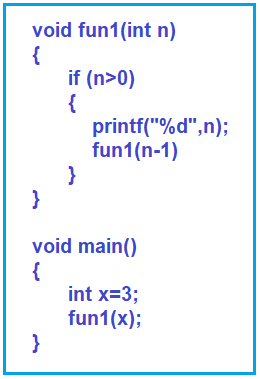As shown in the above example, we have two functions that are fun1() and the main() function. The machine code of these two functions will be there in the code section of the main memory. Now, let us run the program and see how the stack is created.

The program execution starts from the main functions. Inside the main function, int x=3; is the first statement that is X is created. Inside the stack, the activation record for the main function is created and it will have its own variable that is X having value 3.

The very next statement is fun1() i.e. call to the fun1 function. So. once the function fun1() is called, it is having just one variable that is n. The activation record for that fun1() function is created and it will have that variable n and the value x is passed to that n variable, so it will store the value 3. For better understanding, please have a look at the below image. This is the first call of the fun1 function.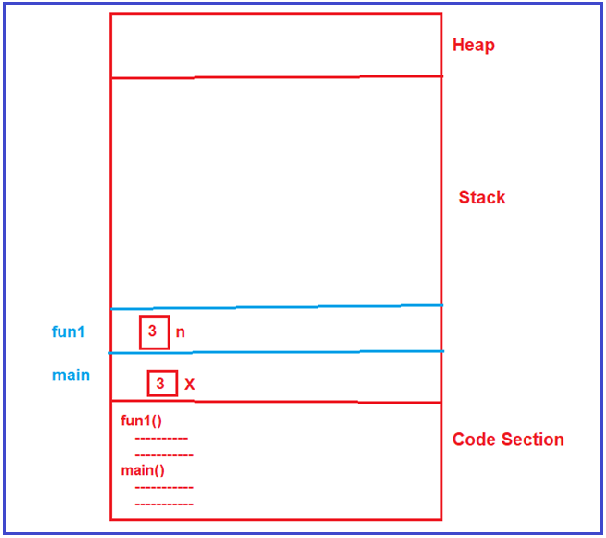Let’s continue. Inside the fun1 function, first, it will check whether n is greater than 0. Yes, n (3) is greater than 0 and the condition satisfies. So, it will print the value 3 and will call the fun1() function with the reduced value of n i.e. n-1 i.e. 2. Once the fun1 function is called, again another activation record for that function is created inside the stack. Within this activation record, again the variable n is created with the value 2 as shown in the below image. This is the second call of the fun1 function.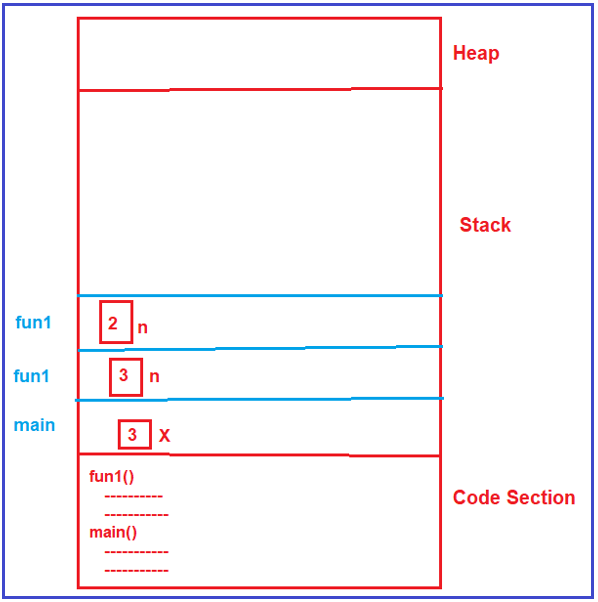In the second call, first, it will check whether n is greater than 0. Yes, n (i.e. 2) is greater than 0 and the condition satisfies. So, it will print the value 2 and will call the fun1() function with the reduced value of n i.e. 2-1 i.e. 1. Once the fun1 function is called, another activation record for that function is created and the variable n is created with the value 1 as shown in the below image. This is the third call of the fun1 function.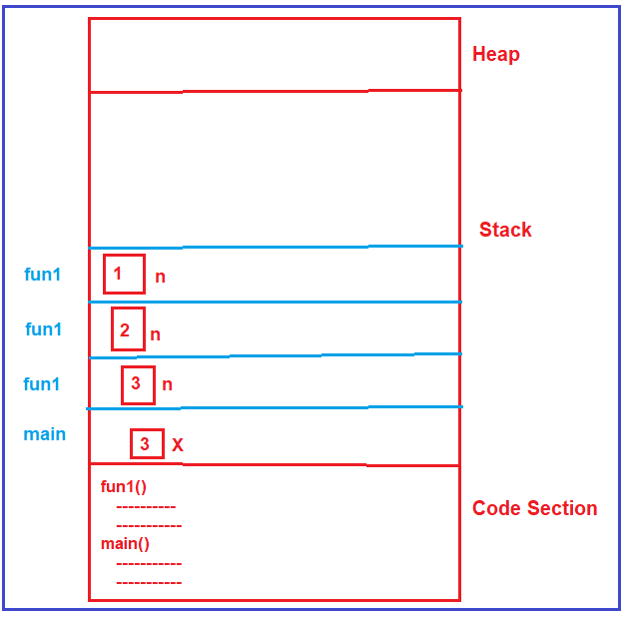In the third fun1 function call, it will check whether n is greater than 0. Yes, n (i.e. 1) is greater than 0. So, it will print the value 1 and again it will call the fun1() function with the reduced value of n i.e. 1-1 i.e. 0. Once the fun1 function is called, again another activation record for the fun1 function is created and the variable n is created with the value 0 as shown in the below image. This is the fourth call of the fun1 function.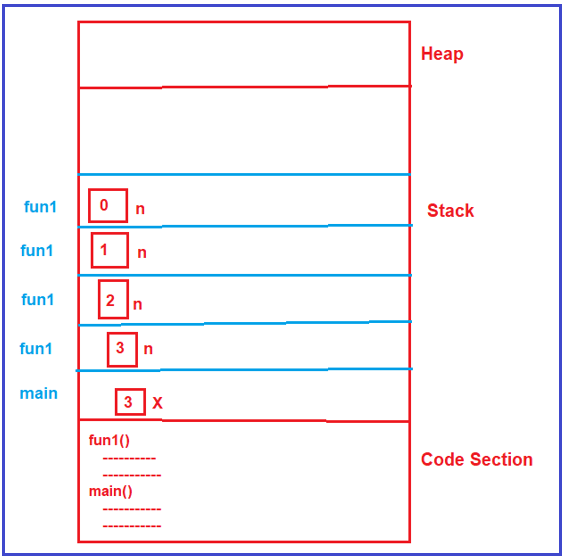Now, in the fourth fun1 function call, it will check whether n is greater than 0. No, n (i.e. 0) is not greater than 0. So, it will not come inside the condition and it will not execute those two statements and it simply comes out of the function. Once the fourth fun1 function call is completed, it will delete that fourth fun1 activation area from the stack as shown in the below image.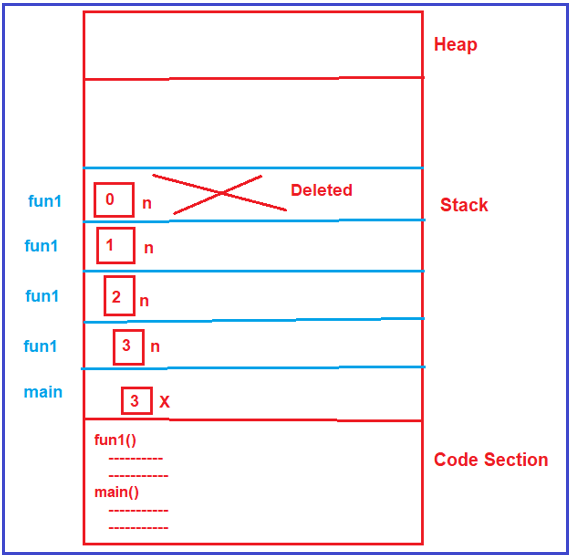Once that function call is completed and once that activation record is deleted from the stack, the control goes back to the previous function call i.e. fun1(1) i.e. the third function call. In the third fun1 function call, there are no more operations to perform, so it simply comes out from that function to the previous function call and also deletes the activation record from the stack as shown in the below image which is created for the third function call.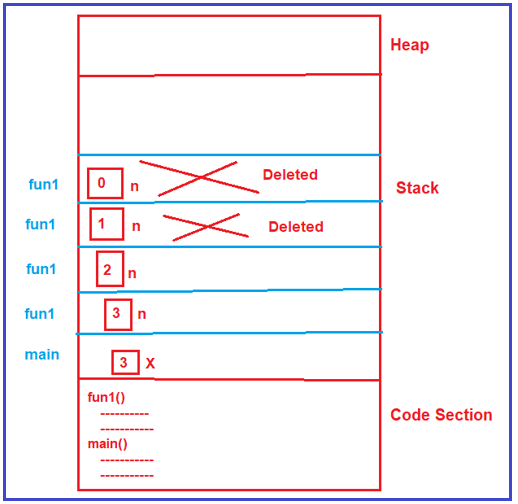Once that activation record is deleted from the stack, the control goes back to the previous function call i.e. fun1(2) i.e. the second function call. In the second fun1 function call, there are no more operations to perform, so it simply comes out from that function to the previous function call and also deletes the activation record from the stack which is created for the second function call as shown in the below image.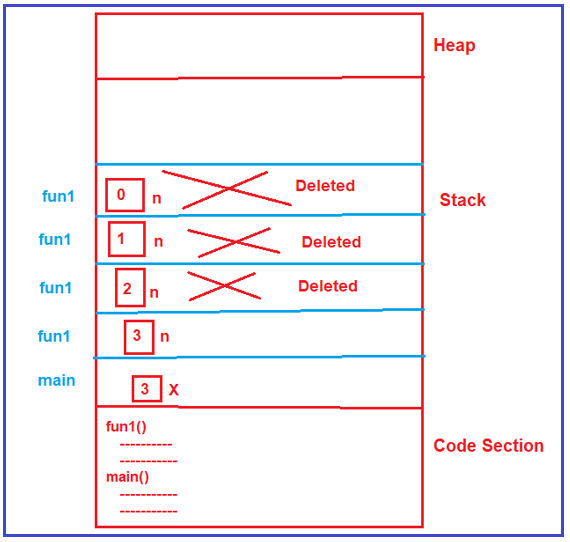Once that activation record for the second function call is deleted from the stack, the control goes back to the previous function call i.e. fun1(3) i.e. the first function call. In the first fun1 function call, there are no more operations to perform, so it simply comes out from that function to the main function and also deletes the activation record from the stack which is created for the first function call as shown in the below image.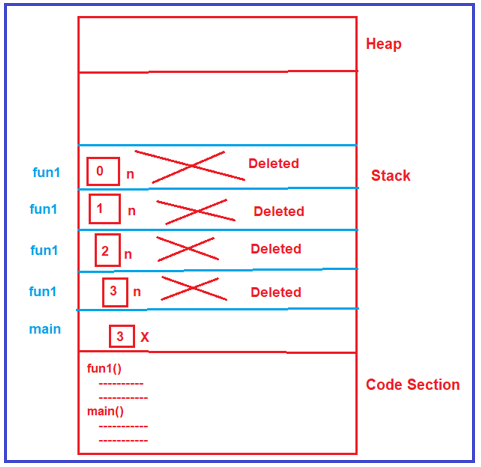Inside the main function after the fun1 function call, there is nothing, so it will also delete the activation record which is created for the main function as shown in the below image.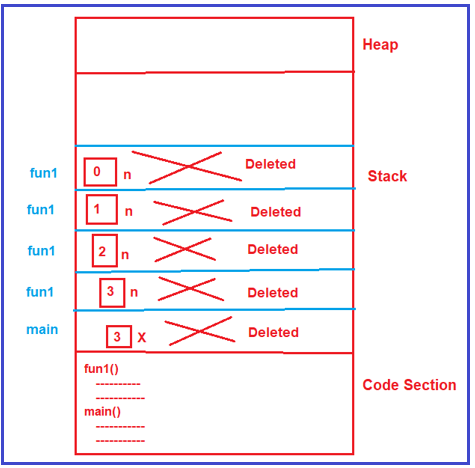This is how a stack is created and utilized a recursion.

##### What is the size of the stack?

Leaving the main function activation record, there are four activation records created for the fun1 function. So, the size of the stack is 4. Each activation record is having just one variable n and we have four activation records. So, the total size is 4 * size of the variable n.

Note: For x = 3, we have four calls. If x = 4, then we have 5 calls. So, for n there will be n+1 calls and also there will be an n+1 activation record.

From this, we understand that recursive functions utilize the stack. Here, internally it takes some extra memory for the stack and hence recursion is a memory-consuming function.

In the next article, I am going to discuss How to find the time complexity of recursive functions in the C Language. Here, in this article, I try to explain How Recursion uses Stack in C Language with Examples and I hope you enjoy this How Recursion uses Stack in C Language with Examples article. Please give your feedback and suggestions about this How Recursion uses Stack in C Programming Language article.